# The Principles of Remote Temperature Measurement

Remote temperature measurement is one of the key application areas for thermopile detectors. This article discusses the fundamental principles of remote temperature measurement.

## Stefan-Boltzman Law

Electromagnetic radiation is emitted by all objects which have a temperature which is above absolute zero (-273.15°C). The Stefan-Boltzman law defines the total amount of power or radiant flux (Φ) released per unit solid angle and per unit area across all wavelengths of radiation.

The Stefan-Boltzman constant is expressed as follows: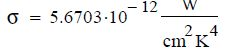The radiance (L) for a Lambertian source is:Where ε is the emissivity of the object surface.

Thermopile detectors produce a voltage in response to thermal energy which is released by any object in its field of view (FOV) and in proportion to the incident power.

This response is termed as the responsivity (R) of the thermopile detector. The responsivity of Dexter Research's model 1M is given as follows: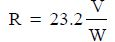The following factors influence the net power exchange between a thermopile and an object (target or source):

• Temperature of the detector (Td) and source (Ts)
• Area , distance, shape an orientation between the source and the detector
• The presence of additional objects in the path such as optics
• The radiative characteristics of surfaces such as emissivity
• Medium such as moisture and atmosphere between the object and the detector

For example, the following values are used in the case of a circular detector and circular source that are placed in parallel to each other and have a common optical axis (Figure 1), where the detector’s FOV is not filled by the source:

• The source radius, rs = 10.6/2cm
• The detector radius, rd = 1.0/2cm
• Emissivity of the source, εs = 1.0
• Emissivity of the detector, εd = 1.0
• The distance between the detector and source, ssd = 10cm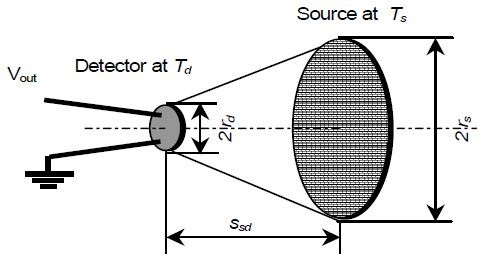Figure 1. Application example

The dimensions of the system in this example are partially included in a "real body view factor", or transfer factor Fsd. The calculations and a catalog of transfer factors for various geometries are provided by Siegel and Howell. The following expression can be used to calculate the Fsd for this example: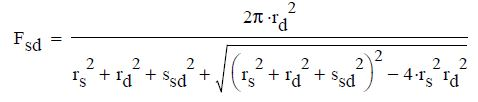The following expression can define the net power exchange through radiation: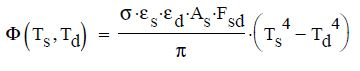Where As is the area of the source and Ad is the area of the detector

If a detector’s active area is square, then a circular detector of equal area should be used, thus yielding a close numerical solution.

If the net power exchange from the source and the responsivity of the detector are known, the following expression can be used to estimate the output signal, Vout: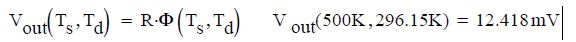Figure 2 presents Vout as a function of Ts for three detector temperatures.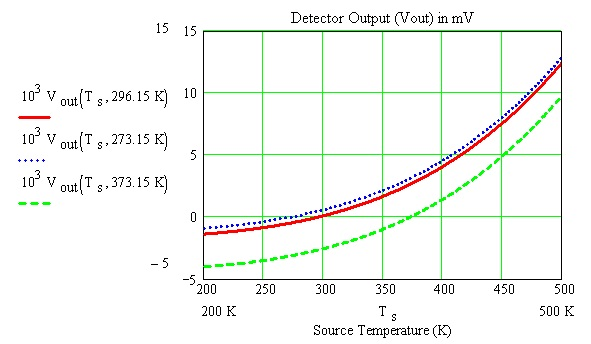Figure 2. Detector output as a function of source temperature

The aforementioned example is a simplified system. However, the solution to the radiant power exchange problem is highly complex in reality. Hence, the following empirical formula can be applied for use in the calibration of an actual instrument: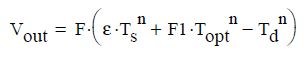Where F and F1 are constants that are based on geometry and Topt is the temperature of the optical components of the radiometer.

The power factor, n, is different due to the limited spectral range of a given radiometer. Temperature sensors such as LM20 from National Semiconductors can be used to monitor the temperatures, Topt and Td. For each individual instrument, a 3-point calibration can be used to determine the constants, F, F1 and n.This information has been sourced, reviewed and adapted from materials provided by Dexter Research.

## Citations

• APA

Dexter Research Center, Inc.. (2019, December 24). The Principles of Remote Temperature Measurement. AZoSensors. Retrieved on November 28, 2020 from https://www.azosensors.com/article.aspx?ArticleID=587.

• MLA

Dexter Research Center, Inc.. "The Principles of Remote Temperature Measurement". AZoSensors. 28 November 2020. <https://www.azosensors.com/article.aspx?ArticleID=587>.

• Chicago

Dexter Research Center, Inc.. "The Principles of Remote Temperature Measurement". AZoSensors. https://www.azosensors.com/article.aspx?ArticleID=587. (accessed November 28, 2020).

• Harvard

Dexter Research Center, Inc.. 2019. The Principles of Remote Temperature Measurement. AZoSensors, viewed 28 November 2020, https://www.azosensors.com/article.aspx?ArticleID=587.